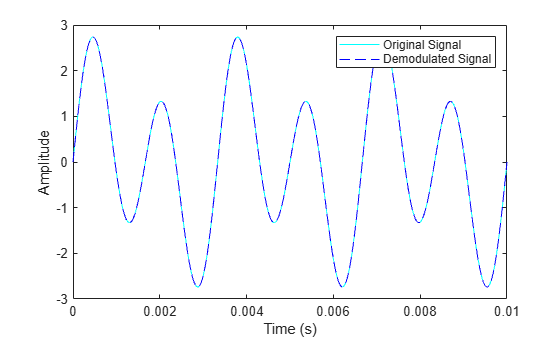Main Content

# amdemod

Amplitude demodulation

## Syntax

``z = amdemod(y,Fc,Fs)``
``z = amdemod(y,Fc,Fs,ini_phase)``
``z = amdemod(y,Fc,Fs,ini_phase,carramp)``
``z = amdemod(y,Fc,Fs,ini_phase,carramp,num,den)``

## Description

````z = amdemod(y,Fc,Fs)` returns a demodulated signal `z`, given the input amplitude modulated (AM) signal `y`, where the carrier signal has frequency `Fc`. The carrier signal and `y` have sampling frequency `Fs`. The modulated signal `y` has zero initial phase and zero carrier amplitude, resulting from a suppressed-carrier modulation. NoteThe value of `Fs` must satisfy `Fs` ≥ 2`Fc`. ```
````z = amdemod(y,Fc,Fs,ini_phase)` specifies the initial phase of the modulated signal in radians.```
````z = amdemod(y,Fc,Fs,ini_phase,carramp)` demodulates a signal created through transmitted-carrier modulation instead of suppressed-carrier modulation, where `carramp` is the carrier amplitude of the modulated signal.```

example

````z = amdemod(y,Fc,Fs,ini_phase,carramp,num,den)` specifies the numerator and denominator of the lowpass Butterworth filter used in the demodulation. The numerator and denominator are generated by [`num`,`den`] = `butter(n,Fc*2/Fs)`, where `n` is the order of the lowpass filter.```

## Examples

collapse all

Set the carrier frequency to 10 kHz and sampling frequency to 80 kHz. Generate a time vector having a duration of 0.01 s.

```fc = 10e3; fs = 80e3; t = (0:1/fs:0.01)';```

Create a two-tone sinusoidal signal with frequencies 300 and 600 Hz.

`s = sin(2*pi*300*t)+2*sin(2*pi*600*t);`

Create a lowpass filter.

`[num,den] = butter(10,fc*2/fs);`

Amplitude modulate the signal `s`.

`y = ammod(s,fc,fs);`

Demodulate the received signal.

`z = amdemod(y,fc,fs,0,0,num,den);`

Plot the original and demodulated signals.

```plot(t,s,'c',t,z,'b--') legend('Original Signal','Demodulated Signal') xlabel('Time (s)') ylabel('Amplitude')```The demodulated signal is nearly identical to the original signal.

## Input Arguments

collapse all

Amplitude modulated input signal, specified as a scalar, vector, matrix, or 3-D array. Each element of `y` must be real.

Data Types: `double` | `single`

Carrier signal frequency in hertz (Hz), specified as a positive scalar.

Data Types: `double`

Sampling frequency of the carrier signal and input message signal in hertz (Hz), specified as a positive scalar. To avoid aliasing, the value of `Fs` must satisfy `Fs` > 2(`Fc` + `BW`), where `BW` is the bandwidth of the original modulated signal.

Data Types: `double`

Initial phase of the modulated signal in radians, specified as a scalar.

Data Types: `double`

Carrier amplitude of the modulated signal, specified as a scalar.

Data Types: `double`

Lowpass Butterworth filter numerator, specified as a scalar.

Data Types: `double`

Lowpass Butterworth filter denominator, specified as a scalar.

Data Types: `double`

## Output Arguments

collapse all

Amplitude demodulated output signal, returned as a scalar, vector, matrix, or 3-D array.

## See Also

### Topics

Introduced before R2006a

## Support

#### Bridging Wireless Communications Design and Testing with MATLAB

Download white paper